Next: About this document ... Up: lab_template Previous: lab_template

Subsections

# MA 1024 Lab 2: Curve Computations in Three Dimensions

## Purpose

The purpose of this lab is to introduce you to curve computations using Maple for parametric curves and vector-valued functions in three dimensions.

## Getting Started

To assist you, there is a worksheet associated with this lab that contains examples. You can copy that worksheet to your home directory with the following command, which must be run in a terminal window, not in Maple.

cp ~bfarr/Curves3D_start.mws ~


You can copy the worksheet now, but you should read through the lab before you load it into Maple. Once you have read to the exercises, start up Maple, load the worksheet Curves3D_start.mws, and go through it carefully. Then you can start working on the exercises

## Background

A parametric curve in three dimensions is a triple of functions
,
,for
in some interval. A vector-valued function in three dimensions is a function
that associates a vector in the plane with each value ofin its domain. Such a vector valued function can always be written in component form as follows,
where
,
, and
are functions defined on some interval. From our definition of a parametric curve, it should be clear that you can always associate a parametric curve with a vector-valued function by just considering the curve traced out by the head of the vector. However, there are lots of situations where a vector-valued function is more appropriate. We will focus on the case of motion of a particle in three dimensions. That is, we have a vector-valued functionthat gives the position at timeof a moving point. The velocity of this point is given by the derivativeand the acceleration is given by the second derivative,
. If the velocity,, is never zero, then we can define the unit tangent vectorand the curvature
the same way we did in two dimensions byandIf the curvature is never zero for a particular curve, then we can define another intrinsic property of curve, the unit normal vector
by the following equation.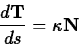It can be shown that at each point on the curve the vectordefined by this equation is a unit vector that is always perpendicular to the tangent vector
at that point. Furthermore, the unit normal vectoralways points in the direction of the centripetal acceleration required to keep a particle moving on the curve. One way to see this is to compute the acceleration by differentiating both sides of the equation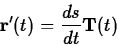Using the chain rule and the definition of the curvature and the normal vector one obtains the following important equation.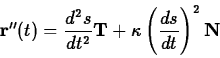To see why this equation is useful, recall that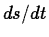is the speed, so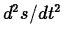is the rate of change of the speed. That is, this term measures whether the particle is speeding up or slowing down. Because this component of the acceleration is in the direction of the tangent vector it is often called the tangential acceleration, denoted by the symbol. The component of the acceleration in the direction of the normal vector is called the normal acceleration, denoted. In the case of motion on a circular path, the curvature is the reciprocal of the radius, so this term should be easily recognizable as the centripetal acceleration.

Computing these quantities is generally not an easy task. The Getting started worksheet for this lab describes commands from the CalcP package that simplify these calculations and provides examples for you to work from.

## Exercises

1. As discussed in the text, the curve associated with a function of the form
whereis a positive constant andis an arbitrary constant, is called a circular helix.
1. Compute the velocity and acceleration for this curve. Using the VDiff command is probably the easiest way.
2. Show that the speed is constant. That is, show that the speed is independent of.
3. Compute the curvature of the circular helix.
2. Consider the function
1. Plot the graph of this function. A good interval is.
2. Do you think the name figure-eight helix is appropriate for this curve? Why or why not?
3. Compute the speed. Is it constant?
4. Using a plot of the curvature over the interval, estimate the maximum value of the curvature.Next: About this document ... Up: lab_template Previous: lab_template
William W. Farr
2003-03-26